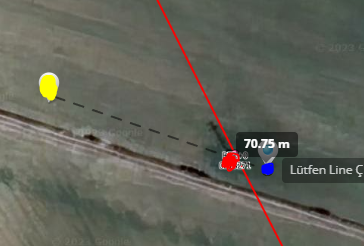# Calculating position of an object.

Hello,

We have an dji matricies 300 rtk video. I would like to detect position of something. Here an example photo what I would like to detect. Green rectangle is my target.Black rectangle is mid point of the image, so focus point of the camera.

First I find some informations like height and position of drone, pitch and yaw angle of drone, compass angle. Then I calculate distance between drone and object like below.

``````distance = OSD.height* tan(GIMBAL.pitch)
``````

With the distance, I use this post to calculate position of the target.

However, there is little bit more error when I compare it with Lidar Laser Range Finder.yellow one is calculation, red one is actual position and blue one is laser range finder. I think calculation an laser range finder should be almost same because both of them measure distance for same point which is center of camera. Is there anything that I do wrong? Here parameters:

drone latitude: 37.4534739401493
drone longitude: 38.6699858861775
drone height [m]: 129.17424
gimbal pitch: -20.1
gimbal yaw  = 326.3
distance between drone and target 352.98

actual object latitude: 37,4560140571369
actual object longitude: 38,6684240400208

calculated object latitude: 37.45611493462226
calculated object longitude: 38.66776707137195

my python function:

``````def calculate_object_position(latitudeDegrees, longitudeDegrees, distance, brng):